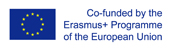This project has been funded with support from the European Commission.
This web site reflects the views only of the author, and the Commission cannot be held responsible for any use which may be made of the information contained therein.

Select language   >   IT EN RO PL LT

### Percentages

Subject taught
- Math

Type of Product
- Web Site/Portal

Language Skills Developed

Transferable/Scientific Skills Developed
Student will develop self-motivation, analytical skills, problem-solving skills.

Description
General aims:
Student will be able to do basic mathematical operations with percentages which can be used in the real life. He will know basic vocabulary in English connected with percentages. Student will develop positive learning habits such as organisational and problem-solving skills.

Linguistic aims:
- to read English with ease
- to understanding content
- to learn from written texts
- to master tools for gathering information
- to express understanding and experiences
- to enable students to understand topic correctly
- to translate words connected with percentages in English into the mother tongue.

Subject specific aims:
- student knows the definition of percent
- student is able to express a percent as a fraction or as a decimal
- student knows what the difference between percent and percentage is
- student can calculate percentages using fractions
- student can solve basic word problems using percentages.

Target group age:
Students from primary school (12-13 years old), before using the resource they need to know how to do operation with fractions and decimals. What is more, this web site can be used by anyone who wants to do basic mathematical operations with percents.

Level of competence in English (CEFR)
B1/B1+

Time required to use the resource with the students:
The material can be divided into two parts. This way the teacher can use the resource with the students for two lessons.

How to use it
The teacher can ask the students to familiarize themselves with the website at home without the part called “Some Worked Examples”. Students should try to understand the text in English as well as the concept of percent and the content of the website.

During lessons the teacher can ask the student in English:
“What is the percent?”,
“What is the origin of this word?”,
“How to express a percent as a fraction or as a decimal?”
“How to calculate the percent of the different numbers?”.
The students give the answers with the examples. The teacher should point the good answers on the web site and show students the examples from the resource to visualize the most important definitions and operations. The teacher highlights some new words for students in English and students should find the translation these words into the mother tongue and write down the new words in English in the notebook with the right translation.
Then, the students do the exercises for example: colouring in the correct percentage of the number square, expressing a percent as a fraction or as a decimal, calculating the percent of the different numbers. For the last task the teacher can use the slider which is on the web site in order to check students’ answers.
The second classes can start with the introduction of “Some Worked Examples” from the resource. The students can discussed them in pairs and after that tried to express their conclusions and possible questions. The teacher comments and corrects their statements and shows them the “Calculation Trick” which is introduced on the web site. Then the student can solve math problems which are on the website in the part called “Your turn.” After doing and checking the exercises teacher can ask students what the difference between percent and percentage is. If they do not know the answer they can find it on the web site (Percentage vs Percentage).
The teacher can recommend and encourage the students to get to know more about percentages using other pages of the resource (“here’s more”).
Possible difficulties for the students
Students who have poor knowledge of English can have problems with understanding the content. Students who has problems doing basic operations with fractions and decimals can face difficulties in solving math problems with percentages which are introduced on the web site which is recommended.